# 추천시스템에 사용되는 metrics 정리

## Intro

기계학습, 딥러닝 분야에서는 모델의 성능을 측정하는 수많은 metric들이 존재합니다. 대표적으로는 정확도(accuracy), AUC score 등이 있습니다. 하지만 이러한 metric들은 추천시스템에 바로 적용하기에는 무리가 있습니다. 왜냐하면 추천시스템은 두 가지 질문에 대답할 수 있는 metric을 찾아야하기 때문입니다.

1. 추천 시스템이 사용자가 선호하는 아이템을 얼마나 상위권에 잘 올려놓았는가?
2. 사용자에게 있어 추천된 아이템 간의 상대적인 선호도가 잘 반영되었는가?

그래서 저는 ‘추천시스템’에서 사용되는 metric에는 어떤 것이 있는지 궁금해졌습니다. 이 글은 해당 포스트를 읽고 덧대어 정리하는 글입니다.

## metrics

추천 문제의 경우 때에 따라 regression 혹은 classification으로 바라볼 수 있습니다. ‘사용자가 해당영화에 몇점의 평점을 내릴까’에 대한 문제를 풀면 regression 문제가 되며, ‘사용자가 해당영화를 좋아할까?’에 대한 문제를 풀면 binary classification이 됩니다. 하지만 대부분의 경우에는 추천 문제를 binary classification문제로 가정하는 것 같습니다. 따라서 기본적으로 classification에서 사용되는 주요 metric들에 대한 개념을 집고가는 것이 좋을 것 같습니다.위와같은 confusion matrix가 있다고 가정하고 설명하도록 하겠습니다. True positive와 같은 네이밍은 <그게 맞앗냐?> <예측한거> 라고 생각하시면 쉽게 외워집니다. 즉, True positive는 Postivie라고 예측하고 True(맞았다)를 의미하며 False Negative는 Negative라고 예측했는데 False(틀렸다 = 즉 Positive인데 Negative로 예측했다)를 의미합니다.

• Accuracy

정확도는 전체 test set에서 긍정과 부정을 포함하여 몇개를 맞았는가로 계산할 수 있습니다. 따라서 식으로는 다음과 같이 표현할 수 있습니다. $\frac{(TP+TF)}{(TP+TF+FP+FN)}$ 전체 set에서 긍정으로 예측했는데 실제로 긍정인 것과 부정으로 예측했는데 실제로 부정인 것의 비율을 의미합니다.

• Precision

precision은 한국어로는 정밀도를 뜻합니다. 모델이 실제로 Positive로 예측한 것 중 실제 Positive인 것의 비율입니다. 식으로는 $\frac{TP}{TP+FP}$로 쓸 수 있습니다. 영화 추천의 문제라고 한다면 실제로 추천한 영화중에 사용자가 선호하는 영화는 얼마나 되었나?를 의미할 수 있습니다. Precision은 P로 시작하고 식도 다 P만 있다고 기억하면 외우기 좋습니다 :)

• Recall

recall은 한국어로 재현율을 의미합니다. 실제 True인 것중에 모델이 True라고 예측한 것의 비율입니다. 식으로는 $\frac{TP}{TP+FN}$로 쓸 수 있습니다. False Negative는 N으로 예측했지만 False이니 실제로는 True를 의미하는 것이겠지요 ^^ 다시 한 번 영화 추천의 문제라고 한다면 실제 사용자가 선호하는 영화를 추천에서 얼마나 잘 맞췄나?를 의미하게 될 것입니다.

• F1

F1 score는 Precision과 Recall을 동시에 고려하는 하나의 metric입니다. precision score와 recall score의 조화평균으로 구할 수 있습니다. 식으로는 $\frac{2 * (precision * recall)}{(precision+recall)}$로 쓸 수 있습니다.

• AUC

AUC score는 ROC curve 의 면적을 구한 값입니다. ROC curve는 True positive rate과 1-True negative rate의 비율을 그래프로 그린 것입니다. threshold에 따른 변화를 기준으로 모델이 가진 classficiation performance를 측정하는 좋은 metric입니다. 자세한 설명을 여기서 하면 굉장히 길어지기 때문에 정말 잘 설명해주는 좋은 글을 추천하는걸로 마무리하겠습니다.

## Rank-less recommendation metrics

그렇다면 위와같은 일반적인 metric을 추천 task에서 잘 사용하지 않는 이유는 무엇일까요? 위의 metric들은 test set의 전체 데이터를 대상으로 성능을 측정하게됩니다. 하지만 추천모델은 단순히 ‘맞았냐 안맞았냐’를 측정하기보단 ‘맞추되 얼마나 상위에 위치시킬 수 있는가?’가 매우 중요합니다. 예를 들어봅시다. 넷플릭스에서 여러분의 취향이라며 영화 2500편을 추천해준다면 사용자로서 기분이 좋을까요? 전혀요. 이처럼 추천모델의 핵심은 상위 k개의 추천안에 실제로 사용자가 좋아할만할 아이템을 넣어야한다는 것입니다. 따라서 흔히 쓰이는 precision, recall은 추천 task에서 precision@k, recall@k라는 변형된 모습으로 사용됩니다. k개의 추천에 대한 평가지표로서 사용되는 것이죠.

지금부터 예시를 통해 각 metric을 어떻게 측정하는지 들여다 보겠습니다.

유저 A와 B가 선호하는 (relevant) 영화는 ‘기생충’, ‘범죄와의 전쟁’, ‘아바타’, ‘테넷’입니다. 실제로 각 유저들이 선호하는 영화는 각각 다르겠지만 여기서는 편리성을 위해 둘 다 선호하는 영화가 같다고 가정하겠습니다. 우리의 추천모델은 유저 A에게 3개의 영화를 추천하였는데 ‘7광구’, ‘범죄와의 전쟁’, ‘기생충’를 추천하였습니다. 유저 B에게 역시 3개의 영화를 추천하는데 ‘아저씨’, ‘공동경비구역 JSA’, ‘아바타’를 추천하였습니다.

• Precision@k

precision@k는 k개의 추천 중 실제로 사용자가 선호하는 (relevant) 아이템이 얼마나 존재하는지를 측정하는 지표입니다. 분모는 추천한 아이템의 개수, 분자는 relevant한 아이템의 개수입니다. 따라서 유저 A의 경우 precision@3 = 2/3, 유저 B의 경우 1/3 의 값을 갖게 됩니다. 여기서 Precision의 의미는 “사용자에게 useful한 item을 얼마나 잘 추천해주었는가”입니다.

• Recall@k

recall@k는 전체 relevant한 아이템 중 추천된 아이템이 속한 비율입니다. 분모는 전체 relevant한 아이템의 개수, 분자는 k개의 추천 중 relevant한 아이템의 개수입니다. 따라서 유저 A의 경우 recall@3 = 2/4, 유저 B의 경우 1/4의 값을 갖게 됩니다. 왜냐하면 유저 A에게 추천한 아이템 3개 중 실제 relevant한 아이템은 2개, 유저 B의 경우 1개이기 때문이지요. 여기서 recall의 의미는 “useful stuff에 대한 추천을 최대한 놓치지 않는 것”입니다.

• Hit@k

때로는 hit@k를 사용하기도 합니다. k개의 추천 중에 relevant한 것이 있으면 1 아니면 0입니다. 따라서 유저 A의 경우 1, 유저 B의 경우도 1입니다.

어찌되었든 결과적으로는 해당 metric들을 모든 user로부터 구한 뒤 user 수로 평균을 내서 test set에 대한 metric을 구할 수 있습니다. 위 예시에서는 precsion@3은 (2/3 + 1/3) / 2 = 1/2, recall@3은 (2/4 + 1/4) / 2 = 3/8, hit@3은 (1 + 1) / 2 = 1 이겠지요.

## Rank-aware recommendation metrics

위에서의 Rank-less metric의 경우 rank에 따른 상대적인 선호도를 제대로 반영하지 못한다는 단점이 있습니다. 즉, 똑같은 relevant 아이템이라고 하더라도 이를 추천 결과의 첫 번째에서 추천하는 모델과 열 번째에서 추천하는 모델에는 분명히 성능차이가 존재한다고 할 수 있습니다. 위의 예시에서 유저B 에게 ‘7광구’, ‘범죄와의 전쟁’, ‘기생충’의 순서로 영화를 추천하나 ‘기생충’, ‘7광구’, ‘범죄와의 전쟁’의 순서로 추천하나 metric에는 변화가 없었습니다. 사용자가 좋아하는 ‘기생충’이 더 상위에 있는 모델이 좋은 모델이 아닐까요? 이전에 말했던 것과 같이 사용자들은 자신의 추천목록을 보기 위해 200번의 스크롤을 내리거나 하지는 않으니까요 ^^ 그래서 아래의 metric들이 빛을 발하고 있습니다. 추천된 상대적인 위치에 따라서 점수를 차등하여 주고 있는 점이 특징입니다. 위의 예시를 그대로 사용하여 metric을 살펴보도록 하겠습니다.

유저 A와 B가 선호하는 (relevant) 영화는 ‘기생충’, ‘범죄와의 전쟁’, ‘아바타’, ‘테넷’입니다. 실제로 각 유저들이 선호하는 영화는 각각 다르겠지만 여기서는 편리성을 위해 둘 다 선호하는 영화가 같다고 가정하겠습니다. 우리의 추천모델은 유저 A에게 3개의 영화를 추천하였는데 ‘7광구’, ‘범죄와의 전쟁’, ‘기생충’를 추천하였습니다. 유저 B에게 역시 3개의 영화를 추천하는데 ‘아저씨’, ‘공동경비구역 JSA’, ‘아바타’를 추천하였습니다.

• MRR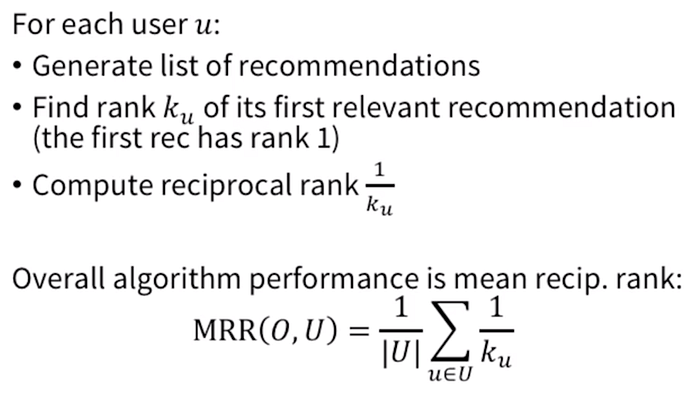MRR은 Mean Reciprocal Rank의 약자입니다. Reciprocal rank는 첫 번째로 등장하는 relevant한 아이템이 우리의 추천상 몇 번째에 위치하는지를 나타내는 지표입니다. 하지만 그대로 몇 번째인지를 사용하면 앞에 나올 수록 좋은 모델이라는 것을 반대로 표현하게되니 역수를 취해주게 됩니다. 유저 A의 경우 relevant 아이템이 두 번째 (범죄와의 전쟁)로 나타났으니 1/2입니다. 유저 B의 경우 relevant 아이템이 세 번째 (기생충)로 나타났으니 1/3입니다. 따라서 이를 평균내준 (1/2 + 1/3) / 2 = 5/12가 MRR이 됩니다.

위의 예시와는 다르지만 이해를 돕기위한 그림을 가져와보면 아래와 같습니다.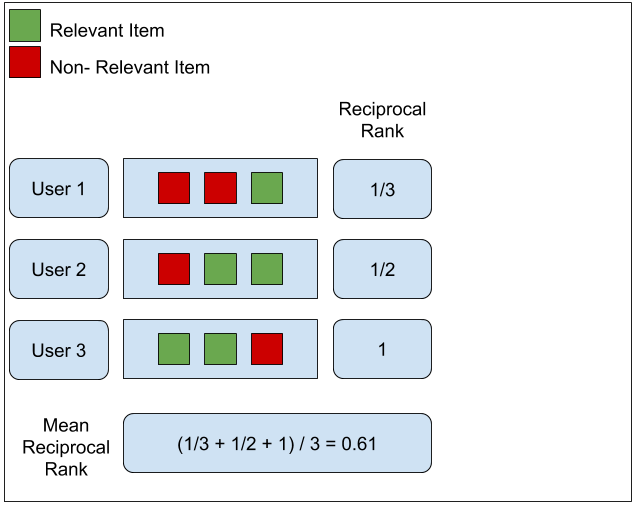하지만 MRR은 추천 상의 몇 개의 relevant아이템이 나오든 첫 번째로 나오는 relevant 아이템만 신경쓴다는 단점을 가지고있습니다. 사용자는 단 하나의 성공적인 추천을 원하는 것이 아니라 추천 리스트 안에서 이 아이템 저 아이템을 비교해볼 수 있습니다.

• MAP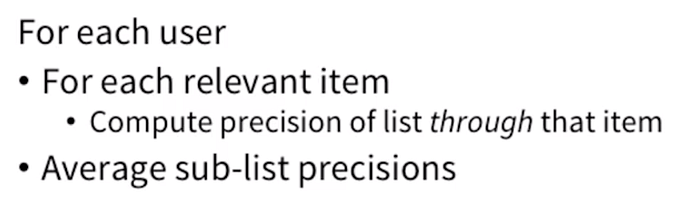MAP는 Mean Average Precision의 약자입니다. Average Precision은 precision@k에서 k를 점점 늘려가며 얻게되는 precision score를 평균낸 것입니다. 추천의 대상들을 ordered list라고 생각하고 첫 번째 추천부터 차례로 내려가며 relevant 아이템이 나올때마다 precision을 구해서 평균내는 것입니다. 유저 A의 경우로 살펴보겠습니다. ‘7광구’, ‘범죄와의 전쟁’, ‘기생충’의 추천리스트에서 7광구는 첫 번째 아이템이지만 relevant하지 않기 때문에 측정하지 않습니다. 이후 두 번째(2) 범죄와의 전쟁은 relevant하므로 1/2 (7광구, 범죄와의전쟁 중 범죄와의 전쟁이 relevant), 마지막으로 세 번째(3) 기생충의 경우 역시 relevant하므로 2/3 (7광,범죄,기생 중 범죄,기생)을 더한 뒤 평균인 (1/2 + 2/3) / 2 = 7/12가 average precision이 되는 것입니다. 이후 ‘Mean’이 의미하는 것처럼 모든 사용자에 대해 Average precision을 구해 평균내면 MAP를 구할 수 있게 됩니다.

마찬가지로 이해를 돕는 그림을 첨부하겠습니다.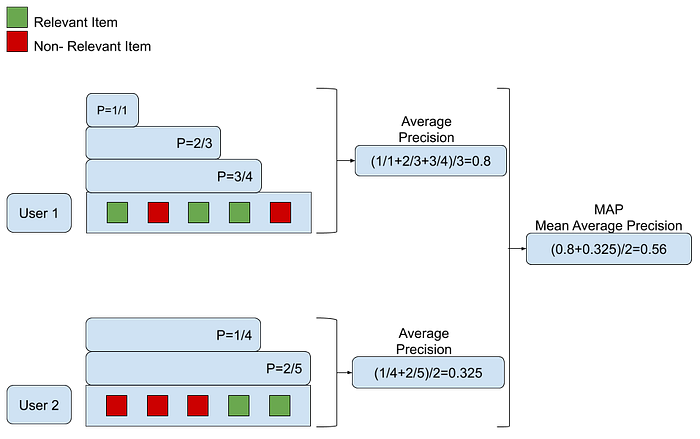MAP는 여러 가지 relevant한 아이템들을 추천 내에서 상대적인 score로 반영할 수 있다는 큰 장점이 있습니다. 하지만 이 metric은 binary rating (1/0)에 최적화되어있습니다. 1~5점으로 star를 매기는 rating과 같은 경우에는 적용할 수 없다는 단점이 있습니다.

• nDCG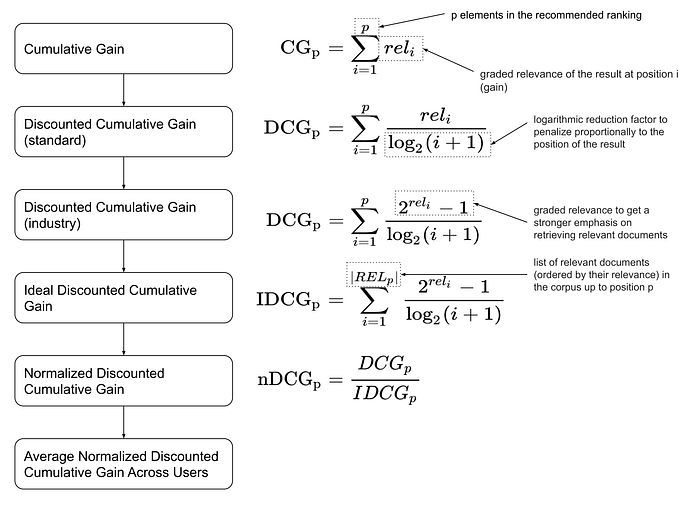여기서 rel_i는 i번째 위치에 추천된 아이템의 relevance score입니다. 예를들어 유저 A에게 relevant한 아이템은 ‘기생충’, ‘범죄와의 전쟁’, ‘아바타’, ‘테넷’이지만 각각 생각하는 선호에는 차이가 있을 수 있습니다. 예컨대 기생충은 5점, 범죄와의 전쟁은 3점, 아바타는 4점, 테넷은 5점과 같이 말이지요. 이전 MRR과 MAP가 단순히 1,0으로 분리했던것과는 달리 구체적인 rating으로 선호를 표현할 수 있습니다. (물론 비선호 아이템에 대한 score는 0점입니다)

nDCG는 normalized Discounted Cumulative Gain의 약자입니다. 차근차근 개념을 쌓아가야하는데 먼저 CG(Cumulative Gain)의 개념은 아주 쉽습니다. relevance score를 그냥 더하는 것입니다. (relevance score라는 말은 편의를 위한 말일 뿐 명확한 용어가 아닙니다) 유저 A의 경우 0(7광구) + 3(범죄와의전쟁) + 5(기생충) = 8이 CG의 값이 됩니다. 하지만 이렇게 CG를 구하게 되면 Rank-less한 metric과 크게 다르지 않습니다. 선호하는 아이템이 어디에 위치하느냐에 따라 이를 조정해주어야하는데 이 때 바로 Discount 개념이 도입됩니다. relevance score를 등장 위치에 따라 조정하는 것입니다. 일반적인 DCG는 log2 를 베이스로하여 Discount가 일어나게 됩니다. 다시 한 번 유저 A의 경우 DCG를 구하면 0 + 3 / $log_{2}(2+1)$ + 5 / $log_{2}(3+1)$ = 약 4.39가 됩니다.

이로써 relevance score의 값과 순위를 모두 반영한 DCG라는 metric을 구할 수 있습니다. 하지만 위 metric의 경우 한계점이 있는데 바로 이 k개의 추천 개수가 커지면 커질수록 점수가 높아진다는 것입니다. k에 따라 고무줄처럼 metric 값이 늘었다 줄었다 할 수 있는 치명적인 단점이 있는 것이지요. 따라서 이를 normalize (0~1) 하는 과정이 필요하게 됩니다. 이 때 사용되는 것이 IDCG (Ideal Discounted Cumulative Gain)인데 이미 주어진 추천의 가장 이상적인 상태를 DCG로 구한 것입니다. 예를들어 우리 모델이 유저 A에게 똑같은 아이템을 추천한다고 할 때 이상적인 모습은 어떤 것인가요? 바로 ‘기생충’, ‘범죄와의 전쟁’, ‘7광구’의 순서입니다. 왜냐하면 유저 A가 생각하는 선호 순서대로 추천하는 모습이 가장 이상적이기 때문입니다. 이 때의 DCG score를 구한 것이 바로 IDCG입니다. 식으로는 5 / $log_{2}(1+1)$ + 3 / $log_{2}(2+1)$ + 0 = 6.89가 되겠네요.

따라서 식으로 nDCG를 구하게 되면 4.39/6.89인 0.6372를 구할 수 있습니다. 0~1의 일정한 범위를 가지며 상대적인 선호도를 고려하는 metric을 구할 수 있게 되었습니다.

## conclusion

이렇게 추천에서 활용 가능한 여러 가지 metric에 대해 알아봤습니다. 추천 task와 관련된 논문을 보며 추천 리스트에 대한 평가를 한다는 것이 감이 오지 않았는데 정리를 해보니 확실히 도움이 되었습니다. 제가 혹시 글에 오류나 오탈자를 적어놨으면 알려주시면 감사드리겠습니다!!

Categories:

Updated: